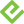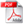# Statistical Analysis: Microsoft Excel 2010

### eBook (Watermarked)

• List Price: \$31.99
• Includes EPUB, MOBI, and PDF
• This eBook includes the following formats, accessible from your Account page after purchase:EPUB The open industry format known for its reflowable content and usability on supported mobile devices.MOBI The eBook format compatible with the Amazon Kindle and Amazon Kindle applications.PDF The popular standard, used most often with the free Adobe® Reader® software.

This eBook requires no passwords or activation to read. We customize your eBook by discreetly watermarking it with your name, making it uniquely yours.

## Description

• Dimensions: 7" x 9-1/8"
• Edition: 1st
• eBook (Watermarked)
• ISBN-10: 0-13-268190-0
• ISBN-13: 978-0-13-268190-2

Statistical Analysis: Microsoft Excel 2010

“Excel has become the standard platform for quantitative analysis. Carlberg has become a world-class guide for Excel users wanting to do quantitative analysis. The combination makes Statistical Analysis: Microsoft Excel 2010 a must-have addition to the library of those who want to get the job done and done right.”

—Gene V Glass, Regents’ Professor Emeritus, Arizona State University

Use Excel 2010’s statistical tools to transform your data into knowledge

Use Excel 2010’s powerful statistical tools to gain a deeper understanding of your data,
make more accurate and reliable inferences, and solve problems in fields ranging from business to health sciences.

Top Excel guru Conrad Carlberg shows how to use Excel 2010 to perform the core statistical tasks every business professional, student, and researcher should master. Using real-world examples, Carlberg helps you choose the right technique for each problem and get the most out of Excel’s statistical features, including its new consistency functions. Along the way, you discover the most effective ways to use correlation and regression and analysis of variance and covariance. You see how to use Excel to test statistical hypotheses using the normal, binomial, t and F distributions.

Becoming an expert with Excel statistics has never been easier! You’ll find crystal-clear instructions, insider insights, and complete step-by-step projects—all complemented by an extensive set of web-based resources.

•  Master Excel’s most useful descriptive and inferential statistical tools

•  Tell the truth with statistics, and recognize when others don’t

•  Accurately summarize sets of values

•  View how values cluster and disperse

•  Infer a population’s characteristics from a sample’s frequency distribution

•  Explore correlation and regression to learn how variables move in tandem

•  Understand Excel’s new consistency functions

•  Test differences between two means using z tests, t tests, and Excel’s

•  Use ANOVA and ANCOVA to test differences between more than two means

•  Explore statistical power by manipulating mean differences, standard errors, directionality, and alpha

There is an Excel workbook for each chapter, and each worksheet is keyed to one of the book's figures. You'll also find additional material, such as a chart that demonstrates how statistical power shifts as you manipulate sample size, mean differences, alpha and directionality. To access these free files, please visit http://www.quepublishing.com/title/0789747200 and click the Downloads Tab.

## Sample Content

Introduction

Chapter 1 About Variables and Values

Variables and Values

Recording Data in Lists

Scales of Measurement

Category Scales

Numeric Scales

Telling an Interval Value from a Text Value

Charting Numeric Variables in Excel

Charting Two Variables

Understanding Frequency Distributions

Using Frequency Distributions

Building a Frequency Distribution from a Sample

Building Simulated Frequency Distributions

Chapter 2 How Values Cluster Together

Calculating the Mean

Understanding Functions, Arguments, and Results

Understanding Formulas, Results, and Formats

Calculating the Median

Choosing to Use the Median

Calculating the Mode

Getting the Mode of Categories with a Formula

From Central Tendency to Variability

Chapter 3 Variability: How Values Disperse

Measuring Variability with the Range

The Concept of a Standard Deviation

Arranging for a Standard

Thinking in Terms of Standard Deviations

Calculating the Standard Deviation and Variance

Squaring the Deviations

Population Parameters and Sample Statistics

Dividing by N − 1

Bias in the Estimate

Degrees of Freedom

Excel’s Variability Functions

Standard Deviation Functions

Variance Functions

Chapter 4 How Variables Move Jointly: Correlation

Understanding Correlation

The Correlation, Calculated

Using the CORREL() Function

Using the Analysis Tools

Using the Correlation Tool

Correlation Isn’t Causation

Using Correlation

Removing the Effects of the Scale

Using the Excel Function

Getting the Predicted Values

Getting the Regression Formula

Using TREND() for Multiple Regression

Combining the Predictors

Understanding “Best Combination”

Understanding Shared Variance

A Technical Note: Matrix Algebra and Multiple Regression in Excel

Moving on to Statistical Inference

Chapter 5 How Variables Classify Jointly: Contingency Tables

Understanding One-Way Pivot Tables

Running the Statistical Test

Making Assumptions

Random Selection

Independent Selections

The Binomial Distribution Formula

Using the BINOM.INV() Function

Understanding Two-Way Pivot Tables

Probabilities and Independent Events

Testing the Independence of Classifications

The Yule Simpson Effect

Summarizing the Chi-Square Functions

Chapter 6 Telling the Truth with Statistics

Problems with Excel’s Documentation

A Context for Inferential Statistics

Understanding Internal Validity

The F-Test Two-Sample for Variances

Why Run the Test?

Chapter 7 Using Excel with the Normal Distribution

Characteristics of the Normal Distribution

The Unit Normal Distribution

Excel Functions for the Normal Distribution

The NORM.DIST() Function

The NORM.INV() Function

Confidence Intervals and the Normal Distribution

The Meaning of a Confidence Interval

Constructing a Confidence Interval

Excel Worksheet Functions That Calculate Confidence Intervals

Using CONFIDENCE.NORM() and CONFIDENCE()

Using CONFIDENCE.T()

Using the Data Analysis Add-in for Confidence Intervals

Confidence Intervals and Hypothesis Testing

The Central Limit Theorem

Making Things Easier

Making Things Better

Chapter 8 Testing Differences Between Means: The Basics

Testing Means: The Rationale

Using a z-Test

Using the Standard Error of the Mean

Creating the Charts

Using the t-Test Instead of the z-Test

Defining the Decision Rule

Understanding Statistical Power

Chapter 9 Testing Differences Between Means: Further Issues

Using Excel’s T.DIST() and T.INV() Functions to Test Hypotheses

Making Directional and Nondirectional Hypotheses

Using Hypotheses to Guide Excel’s t-Distribution Functions

Completing the Picture with T.DIST()

Using the T.TEST() Function

Degrees of Freedom in Excel Functions

Equal and Unequal Group Sizes

The T.TEST() Syntax

Using the Data Analysis Add-in t-Tests

Group Variances in t-Tests

Visualizing Statistical Power

When to Avoid t-Tests

Chapter 10 Testing Differences Between Means: The Analysis of Variance

Why Not t-Tests?

The Logic of ANOVA

Partitioning the Scores

Comparing Variances

The F Test

Using Excel’s F Worksheet Functions

Using F.DIST() and F.DIST.RT()

Using F.INV() and FINV()

The F Distribution

Unequal Group Sizes

Multiple Comparison Procedures

The Scheffé Procedure

Planned Orthogonal Contrasts

Chapter 11 Analysis of Variance: Further Issues

Factorial ANOVA

Other Rationales for Multiple Factors

Using the Two-Factor ANOVA Tool

The Meaning of Interaction

The Statistical Significance of an Interaction

Calculating the Interaction Effect

The Problem of Unequal Group Sizes

Repeated Measures: The Two Factor Without Replication Tool

Excel’s Functions and Tools: Limitations and Solutions

Power of the F Test

Mixed Models

Chapter 12 Multiple Regression Analysis and Effect Coding: The Basics

Multiple Regression and ANOVA

Using Effect Coding

Effect Coding: General Principles

Other Types of Coding

Multiple Regression and Proportions of Variance

Understanding the Segue from ANOVA to Regression

The Meaning of Effect Coding

Assigning Effect Codes in Excel

Using Excel’s Regression Tool with Unequal Group Sizes

Effect Coding, Regression, and Factorial Designs in Excel

Exerting Statistical Control with Semipartial Correlations

Using a Squared Semipartial to get the Correct Sum of Squares

Using TREND() to Replace Squared Semipartial Correlations

Working with the Residuals

Using Excel’s Absolute and Relative Addressing to Extend the Semipartials

Chapter 13 Multiple Regression Analysis: Further Issues

Solving Unbalanced Factorial Designs Using Multiple Regression

Variables Are Uncorrelated in a Balanced Design

Variables Are Correlated in an Unbalanced Design

Order of Entry Is Irrelevant in the Balanced Design

Order Entry Is Important in the Unbalanced Design

Experimental Designs, Observational Studies, and Correlation

Using All the LINEST() Statistics

Using the Regression Coefficients

Using the Standard Errors

Dealing with the Intercept

Understanding LINEST()’s Third, Fourth, and Fifth Rows

Managing Unequal Group Sizes in a True Experiment

Managing Unequal Group Sizes in Observational Research

Chapter 14 Analysis of Covariance: The Basics

The Purposes of ANCOVA

Greater Power

Bias Reduction

Using ANCOVA to Increase Statistical Power

ANOVA Finds No Significant Mean Difference

Adding a Covariate to the Analysis

Testing for a Common Regression Line

Removing Bias: A Different Outcome

Chapter 15 Analysis of Covariance: Further Issues

Adjusting Means with LINEST() and Effect Coding

Effect Coding and Adjusted Group Means

Multiple Comparisons Following ANCOVA

Using the Scheffé Method

Using Planned Contrasts

The Analysis of Multiple Covariance

The Decision to Use Multiple Covariates

Two Covariates: An Example

9780789747204    TOC    4/6/2011